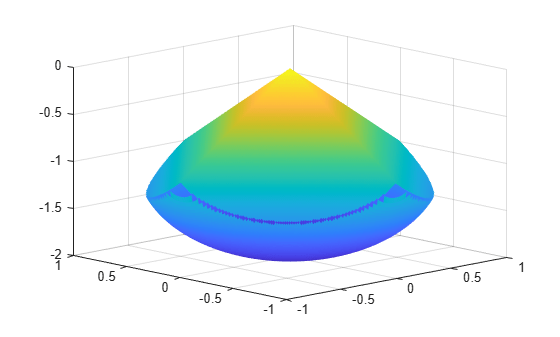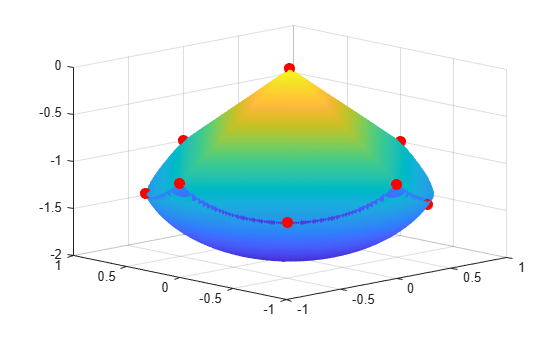# Symbolic Math Toolbox™ Calculates Gradients and Hessians

This example shows how to obtain faster and more robust solutions to nonlinear optimization problems using `fmincon` along with Symbolic Math Toolbox functions. If you have a Symbolic Math Toolbox license, you can easily calculate analytic gradients and Hessians for objective and constraint functions. There are two relevant Symbolic Math Toolbox functions:

• `jacobian` generates the gradient of a scalar function, and generates a matrix of the partial derivatives of a vector function. So, for example, you can obtain the Hessian matrix, the second derivatives of the objective function, by applying `jacobian` to the gradient. Part of this example shows how to use `jacobian` to generate symbolic gradients and Hessians of objective and constraint functions.

• `matlabFunction` generates either an anonymous function or a file that calculates the values of a symbolic expression. This example shows how to use `matlabFunction` to generate files that evaluate the objective and constraint function and their derivatives at arbitrary points.

The syntax and structures of the two sets of toolbox functions differ. In particular, symbolic variables are real or complex scalars, but Optimization Toolbox™ functions pass vector arguments. So there are several steps to take to generate symbolically the objective function, constraints, and all their requisite derivatives, in a form suitable for the `interior-point` algorithm of `fmincon`.

To see the efficiency in using gradients and Hessians, see Compare to Optimization Without Gradients and Hessians. For a problem-based approach to this problem without using derivative information, see Constrained Electrostatic Nonlinear Optimization, Problem-Based.

### Problem Description

Consider the electrostatics problem of placing 10 electrons in a conducting body. The electrons will arrange themselves so as to minimize their total potential energy, subject to the constraint of lying inside the body. It is well known that all the electrons will be on the boundary of the body at a minimum. The electrons are indistinguishable, so there is no unique minimum for this problem (permuting the electrons in one solution gives another valid solution). This example was inspired by Dolan, Moré, and Munson .

This example is a conducting body defined by the following inequalities:

`$z\le -|x|-|y|$`

${x}^{2}+{y}^{2}+\left(z+1{\right)}^{2}\le 1$.

This body looks like a pyramid on a sphere.

```[X,Y] = meshgrid(-1:.01:1); Z1 = -abs(X) - abs(Y); Z2 = -1 - sqrt(1 - X.^2 - Y.^2); Z2 = real(Z2); W1 = Z1; W2 = Z2; W1(Z1 < Z2) = nan; % only plot points where Z1 > Z2 W2(Z1 < Z2) = nan; % only plot points where Z1 > Z2 hand = figure; % handle to the figure, since we'll plot more later set(gcf,'Color','w') % white background surf(X,Y,W1,'LineStyle','none'); hold on surf(X,Y,W2,'LineStyle','none'); view(-44,18)```There is a slight gap between the upper and lower surfaces of the figure. This is an artifact of the general plotting routine used to create the figure. This routine erases any rectangular patch on one surface that touches the other surface.

### Create Variables

Generate a symbolic vector `x` as a 30-by-1 vector composed of real symbolic variables `xij`, `i` between 1 and 10, and `j` between 1 and 3. These variables represent the three coordinates of electron `i`: `xi1` corresponds to the $x$ coordinate, `xi2` corresponds to the $y$ coordinate, and `xi3` corresponds to the $z$ coordinate.

```x = cell(3, 10); for i = 1:10 for j = 1:3 x{j,i} = sprintf('x%d%d',i,j); end end x = x(:); % now x is a 30-by-1 vector x = sym(x, 'real');```

Examine the vector `x`.

`x`
```x =  $\left(\begin{array}{c}{x}_{11}\\ {x}_{12}\\ {x}_{13}\\ {x}_{21}\\ {x}_{22}\\ {x}_{23}\\ {x}_{31}\\ {x}_{32}\\ {x}_{33}\\ {x}_{41}\\ {x}_{42}\\ {x}_{43}\\ {x}_{51}\\ {x}_{52}\\ {x}_{53}\\ {x}_{61}\\ {x}_{62}\\ {x}_{63}\\ {x}_{71}\\ {x}_{72}\\ {x}_{73}\\ {x}_{81}\\ {x}_{82}\\ {x}_{83}\\ {x}_{91}\\ {x}_{92}\\ {x}_{93}\\ {x}_{101}\\ {x}_{102}\\ {x}_{103}\end{array}\right)$```

### Include Linear Constraints

Write the linear constraints

`$z\le -|x|-|y|$`

as a set of four linear inequalities for each electron:

```xi3 - xi1 - xi2 ≤ 0 xi3 - xi1 + xi2 ≤ 0 xi3 + xi1 - xi2 ≤ 0 xi3 + xi1 + xi2 ≤ 0 ```

Therefore there are a total of 40 linear inequalities for this problem.

Write the inequalities in a structured way:

```B = [1,1,1;-1,1,1;1,-1,1;-1,-1,1]; A = zeros(40,30); for i=1:10 A(4*i-3:4*i,3*i-2:3*i) = B; end b = zeros(40,1);```

You can see that `A*x ≤ b` represents the inequalities:

`disp(A*x)`
`$\left(\begin{array}{c}{x}_{11}+{x}_{12}+{x}_{13}\\ {x}_{12}-{x}_{11}+{x}_{13}\\ {x}_{11}-{x}_{12}+{x}_{13}\\ {x}_{13}-{x}_{12}-{x}_{11}\\ {x}_{21}+{x}_{22}+{x}_{23}\\ {x}_{22}-{x}_{21}+{x}_{23}\\ {x}_{21}-{x}_{22}+{x}_{23}\\ {x}_{23}-{x}_{22}-{x}_{21}\\ {x}_{31}+{x}_{32}+{x}_{33}\\ {x}_{32}-{x}_{31}+{x}_{33}\\ {x}_{31}-{x}_{32}+{x}_{33}\\ {x}_{33}-{x}_{32}-{x}_{31}\\ {x}_{41}+{x}_{42}+{x}_{43}\\ {x}_{42}-{x}_{41}+{x}_{43}\\ {x}_{41}-{x}_{42}+{x}_{43}\\ {x}_{43}-{x}_{42}-{x}_{41}\\ {x}_{51}+{x}_{52}+{x}_{53}\\ {x}_{52}-{x}_{51}+{x}_{53}\\ {x}_{51}-{x}_{52}+{x}_{53}\\ {x}_{53}-{x}_{52}-{x}_{51}\\ {x}_{61}+{x}_{62}+{x}_{63}\\ {x}_{62}-{x}_{61}+{x}_{63}\\ {x}_{61}-{x}_{62}+{x}_{63}\\ {x}_{63}-{x}_{62}-{x}_{61}\\ {x}_{71}+{x}_{72}+{x}_{73}\\ {x}_{72}-{x}_{71}+{x}_{73}\\ {x}_{71}-{x}_{72}+{x}_{73}\\ {x}_{73}-{x}_{72}-{x}_{71}\\ {x}_{81}+{x}_{82}+{x}_{83}\\ {x}_{82}-{x}_{81}+{x}_{83}\\ {x}_{81}-{x}_{82}+{x}_{83}\\ {x}_{83}-{x}_{82}-{x}_{81}\\ {x}_{91}+{x}_{92}+{x}_{93}\\ {x}_{92}-{x}_{91}+{x}_{93}\\ {x}_{91}-{x}_{92}+{x}_{93}\\ {x}_{93}-{x}_{92}-{x}_{91}\\ {x}_{101}+{x}_{102}+{x}_{103}\\ {x}_{102}-{x}_{101}+{x}_{103}\\ {x}_{101}-{x}_{102}+{x}_{103}\\ {x}_{103}-{x}_{102}-{x}_{101}\end{array}\right)$`

### Create the Nonlinear Constraints, Their Gradients and Hessians

The nonlinear constraints

`${x}^{2}+{y}^{2}+\left(z+1{\right)}^{2}\le 1$`

are also structured. Generate the constraints, their gradients, and Hessians as follows.

```c = sym(zeros(1,10)); i = 1:10; c = (x(3*i-2).^2 + x(3*i-1).^2 + (x(3*i)+1).^2 - 1).'; gradc = jacobian(c,x).'; % .' performs transpose hessc = cell(1, 10); for i = 1:10 hessc{i} = jacobian(gradc(:,i),x); end```

The constraint vector `c` is a row vector, and the gradient of `c(i)` is represented in the `i`th column of the matrix `gradc`. This is the correct form, as described in Nonlinear Constraints.

The Hessian matrices, `hessc{1}`, ..., `hessc{10}`, are square and symmetric. It is better to store them in a cell array, as is done here, than in separate variables such as `hessc1`, ..., `hesssc10`.

Use the `.'` syntax to transpose. The `'` syntax means conjugate transpose, which has different symbolic derivatives.

### Create the Objective Function, Its Gradient and Hessian

The objective function, potential energy, is the sum of the inverses of the distances between each electron pair:

$energy=\sum _{i.

The distance is the square root of the sum of the squares of the differences in the components of the vectors.

Calculate the energy, its gradient, and its Hessian as follows.

```energy = sym(0); for i = 1:3:25 for j = i+3:3:28 dist = x(i:i+2) - x(j:j+2); energy = energy + 1/sqrt(dist.'*dist); end end gradenergy = jacobian(energy,x).'; hessenergy = jacobian(gradenergy,x);```

### Create the Objective Function File

The objective function should have two outputs, `energy` and `gradenergy`. Put both functions in one vector when calling `matlabFunction` to reduce the number of subexpressions that `matlabFunction` generates, and to return the gradient only when the calling function (`fmincon` in this case) requests both outputs. This example shows placing the resulting files in your current folder. Of course, you can place them anywhere you like, as long as the folder is on the MATLAB path.

```currdir = [pwd filesep]; % You may need to use currdir = pwd filename = [currdir,'demoenergy.m']; matlabFunction(energy,gradenergy,'file',filename,'vars',{x});```

This syntax causes `matlabFunction` to return energy as the first output, and gradenergy as the second. It also takes a single input vector `{x}` instead of a list of inputs `x11`, ..., `x103`.

The resulting file `demoenergy.m` contains, in part, the following lines or similar ones:

```function [energy,gradenergy] = demoenergy(in1) %DEMOENERGY % [ENERGY,GRADENERGY] = DEMOENERGY(IN1) ... x101 = in1(28,:); ... energy = 1./t140.^(1./2) + ...; if nargout > 1 ... gradenergy = [(t174.*(t185 - 2.*x11))./2 - ...]; end ```

This function has the correct form for an objective function with a gradient; see Writing Scalar Objective Functions.

### Create the Constraint Function File

Generate the nonlinear constraint function, and put it in the correct format.

```filename = [currdir,'democonstr.m']; matlabFunction(c,[],gradc,[],'file',filename,'vars',{x},... 'outputs',{'c','ceq','gradc','gradceq'});```

The resulting file democonstr.m contains, in part, the following lines or similar ones:

```function [c,ceq,gradc,gradceq] = democonstr(in1) %DEMOCONSTR % [C,CEQ,GRADC,GRADCEQ] = DEMOCONSTR(IN1) ... x101 = in1(28,:); ... c = [t417.^2 + ...]; if nargout > 1 ceq = []; end if nargout > 2 gradc = [2.*x11,...]; end if nargout > 3 gradceq = []; end ```

This function has the correct form for a constraint function with a gradient; see Nonlinear Constraints.

### Generate the Hessian Files

To generate the Hessian of the Lagrangian for the problem, first generate files for the energy Hessian and for the constraint Hessians.

The Hessian of the objective function, hessenergy, is a very large symbolic expression, containing over 150,000 symbols, as evaluating `size(char(hessenergy))` shows. So it takes a substantial amount of time to run `matlabFunction(hessenergy)`.

To generate a file `hessenergy.m`, run the following two lines:

```filename = [currdir,'hessenergy.m']; matlabFunction(hessenergy,'file',filename,'vars',{x});```

In contrast, the Hessians of the constraint functions are small, and fast to compute:

```for i = 1:10 ii = num2str(i); thename = ['hessc',ii]; filename = [currdir,thename,'.m']; matlabFunction(hessc{i},'file',filename,'vars',{x}); end```

After generating all the files for the objective and constraints, put them together with the appropriate Lagrange multipliers in a file `hessfinal.m`, whose code appears at the end of this example..

### Run the Optimization

Start the optimization with the electrons distributed randomly on a sphere of radius 1/2 centered at [0,0,–1].

```rng default % for reproducibility Xinitial = randn(3,10); % columns are normal 3-D vectors for j=1:10 Xinitial(:,j) = Xinitial(:,j)/norm(Xinitial(:,j))/2; % this normalizes to a 1/2-sphere end Xinitial(3,:) = Xinitial(3,:) - 1; % center at [0,0,-1] Xinitial = Xinitial(:); % Convert to a column vector```

Set the options to use the interior-point algorithm, and to use gradients and the Hessian.

```options = optimoptions(@fmincon,'Algorithm','interior-point','SpecifyObjectiveGradient',true,... 'SpecifyConstraintGradient',true,'HessianFcn',@hessfinal,'Display','final');```

Call `fmincon`.

```[xfinal fval exitflag output] = fmincon(@demoenergy,Xinitial,... A,b,[],[],[],[],@democonstr,options);```
```Local minimum found that satisfies the constraints. Optimization completed because the objective function is non-decreasing in feasible directions, to within the value of the optimality tolerance, and constraints are satisfied to within the value of the constraint tolerance. <stopping criteria details> ```

View the objective function value, exit flag, number of iterations, and number of function evaluations.

`disp(fval)`
``` 34.1365 ```
`disp(exitflag)`
``` 1 ```
`disp(output.iterations)`
``` 19 ```
`disp(output.funcCount)`
``` 28 ```

Even though the initial positions of the electrons were random, the final positions are nearly symmetric.

```for i = 1:10 plot3(xfinal(3*i-2),xfinal(3*i-1),xfinal(3*i),'r.','MarkerSize',25); end``````rotate3d figure(hand)```

### Compare to Optimization Without Gradients and Hessians

The use of gradients and Hessians makes the optimization run faster and more accurately. To compare with the same optimization using no gradient or Hessian information, set the options not to use gradients and Hessians:

```options = optimoptions(@fmincon,'Algorithm','interior-point',... 'Display','final'); [xfinal2 fval2 exitflag2 output2] = fmincon(@demoenergy,Xinitial,... A,b,[],[],[],[],@democonstr,options);```
```Local minimum found that satisfies the constraints. Optimization completed because the objective function is non-decreasing in feasible directions, to within the value of the optimality tolerance, and constraints are satisfied to within the value of the constraint tolerance. <stopping criteria details> ```

Examine the same data as before.

`disp(fval2)`
``` 34.1365 ```
`disp(exitflag2)`
``` 1 ```
`disp(output2.iterations)`
``` 78 ```
`disp(output2.funcCount)`
``` 2463 ```

Compare the number of iterations and number of function evaluations with and without derivative information.

```tbl = table([output.iterations;output2.iterations],[output.funcCount;output2.funcCount],... 'RowNames',{'With Derivatives','Without Derivatives'},'VariableNames',{'Iterations','Fevals'})```
```tbl=2×2 table Iterations Fevals __________ ______ With Derivatives 19 28 Without Derivatives 78 2463 ```

### Clear the Symbolic Variable Assumptions

The symbolic variables in this example have the assumption, in the symbolic engine workspace, that they are real. To clear this assumption from the symbolic engine workspace, it is not sufficient to delete the variables. Clear the variable assumptions by using `syms`:

`syms x`

Verify that the assumptions are empty.

`assumptions(x)`
``` ans = Empty sym: 1-by-0 ```

### Helper Function

The following code creates the `hessfinal` function.

```function H = hessfinal(X,lambda) % % Call the function hessenergy to start H = hessenergy(X); % Add the Lagrange multipliers * the constraint Hessians H = H + hessc1(X) * lambda.ineqnonlin(1); H = H + hessc2(X) * lambda.ineqnonlin(2); H = H + hessc3(X) * lambda.ineqnonlin(3); H = H + hessc4(X) * lambda.ineqnonlin(4); H = H + hessc5(X) * lambda.ineqnonlin(5); H = H + hessc6(X) * lambda.ineqnonlin(6); H = H + hessc7(X) * lambda.ineqnonlin(7); H = H + hessc8(X) * lambda.ineqnonlin(8); H = H + hessc9(X) * lambda.ineqnonlin(9); H = H + hessc10(X) * lambda.ineqnonlin(10); end```

﻿